MathematicsTriangulation and Interpolation of Scattered Data

MATLAB provides routines that aid in the analysis of closest-point problems and geometric analysis.

 Function Description `convhull` Convex hull. `delaunay` Delaunay triangulation. `delaunay3` 3-D Delaunay tessellation. `dsearch` Nearest point search of Delaunay triangulation. `inpolygon` True for points inside polygonal region. `polyarea` Area of polygon. `rectint` Area of intersection for two or more rectangles. `tsearch` Closest triangle search. `voronoi` Voronoi diagram.

This section applies the following techniques to the `seamount` data set supplied with MATLAB:

 Note    Examples in this section use the MATLAB `seamount` data set. Seamounts are underwater mountains. They are valuable sources of information about marine geology. The `seamount` data set represents the surface, in 1984, of the seamount designated LR148.8W located at 48.2°S, 148.8°W on the Louisville Ridge in the South Pacific. For more information about the data and its use, see Parker .The `seamount` data set provides longitude (`x`), latitude (`y`) and depth-in-feet (`z`) data for 294 points on the seamount LR148.8W.

Convex Hulls

The `convhull` function returns the indices of the points in a data set that comprise the convex hull for the set. Use the `plot` function to plot the output of `convhull`.

This example loads the `seamount` data and plots the longitudinal (`x`) and latitudinal (`y`) data as a scatter plot. It then generates the convex hull and uses `plot` to plot the convex hull.

• ```load seamount
plot(x,y,'.','markersize',10)
k = convhull(x,y);
hold on, plot(x(k),y(k),'-r'), hold off
grid on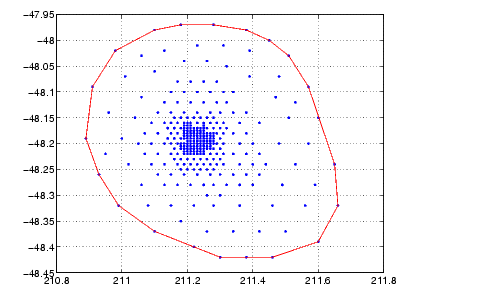```

Delaunay Triangulation

Given a set of coplanar data points, Delaunay triangulation is a set of lines connecting each point to its natural neighbors. The `delaunay` function returns a Delaunay triangulation as a set of triangles having the property that, for each triangle, the unique circle circumscribed about the triangle contains no data points.

You can use `triplot` to print the resulting triangles in two-dimensional space. You can also add data for a third dimension to the output of `delaunay` and plot the result as a surface with `trisurf`, or as a mesh with `trimesh`.

Plotting a Delaunay Triangulation.   To try `delaunay`, load the `seamount` data set and view the longitude (`x`) and latitude (`y`) data as a scatter plot.

• ```load seamount
plot(x,y,'.','markersize',12)
xlabel('Longitude'), ylabel('Latitude')
grid on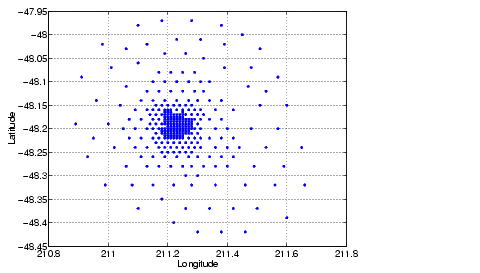```

Apply Delaunay triangulation and use `triplot` to overplot the resulting triangles on the scatter plot.

• ```tri = delaunay(x,y);
hold on, triplot(tri,x,y), hold off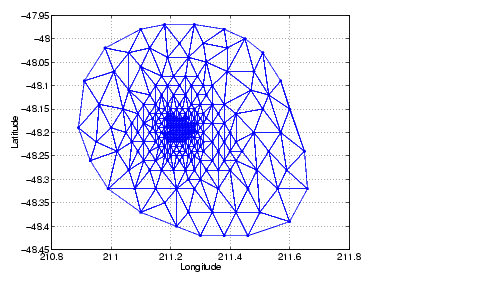```

Mesh and Surface Plots.   Add the depth data (`z`) from `seamount`, to the Delaunay triangulation, and use `trimesh` to produce a mesh in three-dimensional space. Similarly, you can use `trisurf` to produce a surface.

• ```figure
hidden on
trimesh(tri,x,y,z)
grid on
xlabel('Longitude'); ylabel('Latitude'); zlabel('Depth in Feet')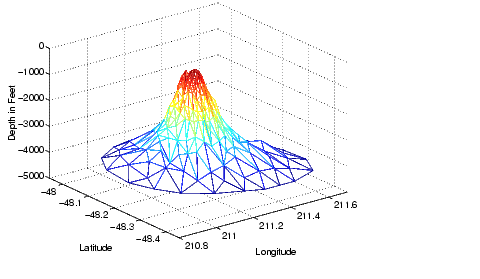```

Contour Plots.   This code uses `meshgrid`, `griddata`, and `contour` to produce a contour plot of the `seamount` data.

• ```figure
[xi,yi] = meshgrid(210.8:.01:211.8,-48.5:.01:-47.9);
zi = griddata(x,y,z,xi,yi,'cubic');
[c,h] = contour(xi,yi,zi,'b-');
clabel(c,h)
xlabel('Longitude'), ylabel('Latitude')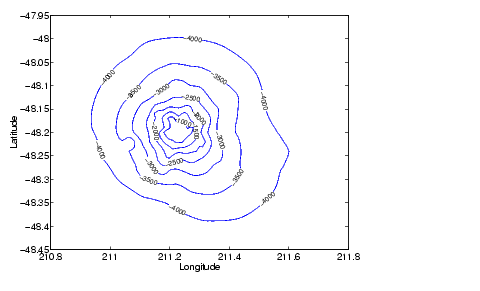```

The arguments for meshgrid encompass the largest and smallest `x` and `y` values in the original `seamount` data. To obtain these values, use `min(x)`, `max(x)`, `min(y)`, and `max(y)`.

Closest-Point Searches.   You can search through the Delaunay triangulation data with two functions:

• `dsearch` finds the indices of the `(x,y)` points in a Delaunay triangulation closest to the points you specify. This code searches for the point closest to (211.32, -48.35) in the triangulation of the `seamount` data.
• ```xi = 211.32; yi = -48.35;
p = dsearch(x,y,tri,xi,yi);
[x(p), y(p)]

ans =
211.3400  -48.3700
```
• `tsearch` finds the indices into the `delaunay` output that specify the enclosing triangles of the points you specify. This example uses the index of the enclosing triangle for the point (211.32, -48.35) to obtain the coordinates of the vertices of the triangle.
• ```xi = 211.32; yi = -48.35;
t = tsearch(x,y,tri,xi,yi);
r = tri(t,:);
A = [x(r) y(r)]

A =
211.3000  -48.3000
211.3400  -48.3700
211.2800  -48.3200
```

Voronoi Diagrams

Voronoi diagrams are a closest-point plotting technique related to Delaunay triangulation.

For each point in a set of coplanar points, you can draw a polygon that encloses all the intermediate points that are closer to that point than to any other point in the set. Such a polygon is called a Voronoi polygon, and the set of all Voronoi polygons for a given point set is called a Voronoi diagram.

The `voronoi` function can plot the cells of the Voronoi diagram, or return the vertices of the edges of the diagram. This example loads the `seamount` data, then uses the `voronoi` function to produce the Voronoi diagram for the longitudinal (`x`) and latitudinal (`y`) dimensions. Note that `voronoi` plots only the bounded cells of the Voronoi diagram.

• ```load seamount
voronoi(x,y)
grid on
xlabel('Longitude'), ylabel('Latitude')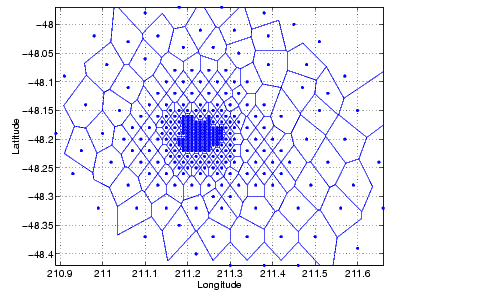```

 Note    See the `voronoi` function for an example that uses the vertices of the edges to plot a Voronoi diagram.Interpolation and Multidimensional Arrays Tessellation and Interpolation of Scattered Data in Higher Dimensions© 1994-2005 The MathWorks, Inc.